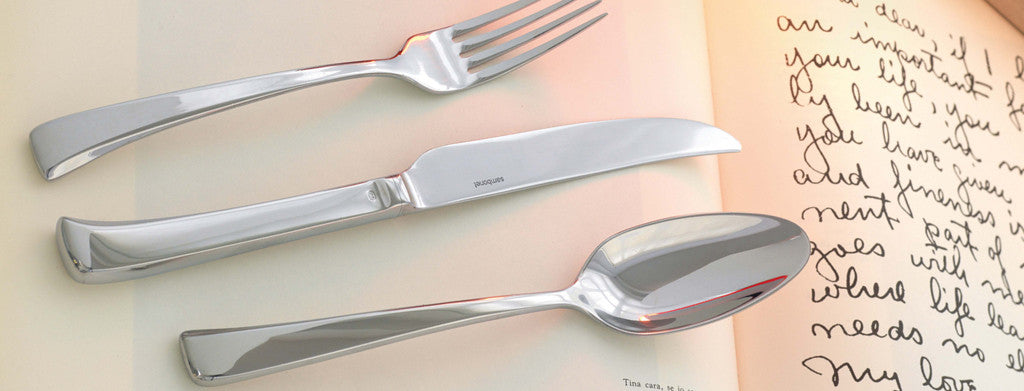Shopping Cart

### Refine

View all

#### Price# Cutlery£8.38 each (ex VAT)£12.01 each (ex VAT)£8.38 each (ex VAT)£4.45 each (ex VAT)£9.17£5.80 each (ex VAT)£29.00£6.15 each (ex VAT)£9.36 each (ex VAT)£6.76 each (ex VAT)£13.90 each (ex VAT)£8.69 each (ex VAT)£35.17 each (ex VAT)£0.34 each (ex VAT)£0.31 each (ex VAT)£1.04 each (ex VAT)£0.55 each (ex VAT)£0.53 each (ex VAT)£0.52 each (ex VAT)£0.97 each (ex VAT)£0.52 each (ex VAT)£1.43 each (ex VAT)£0.76 each (ex VAT)£0.59 each (ex VAT)£0.85 each (ex VAT)£1.30 each (ex VAT)£9.48 each (ex VAT)£4.30 each (ex VAT)£4.30 each (ex VAT)£4.83 each (ex VAT)£6.76 each (ex VAT)£4.83 each (ex VAT)£2.87 each (ex VAT)£4.83 each (ex VAT)£6.26 each (ex VAT)£2.46 each (ex VAT)£3.89 each (ex VAT)£21.74 each (ex VAT)£7.13 each (ex VAT)£1.63 each (ex VAT)£5.40 each (ex VAT)£5.40 each (ex VAT)£2.63 each (ex VAT)£3.26 each (ex VAT)£1.63 each (ex VAT)£21.74 each (ex VAT)£12.59 each (ex VAT)£8.70 each (ex VAT)